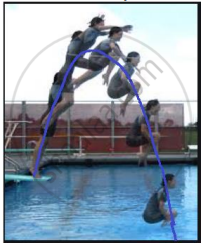# Rita’s height (in feet) above the water level is given by another polynomial p(t) with zeroes -1 and 2. Then p(t) is given by ______. - Mathematics

MCQ
Fill in the Blanks

Case Study -1The figure given alongside shows the path of a diver, when she takes a jump from the diving board. Clearly it is a parabola.

Annie was standing on a diving board, 48 feet above the water level. She took a dive into the pool. Her height (in feet) above the water level at any time‘t’ in seconds is given by the polynomial h(t) such that h(t) = -16t2 + 8t + k.

Rita’s height (in feet) above the water level is given by another polynomial p(t) with zeroes -1 and 2. Then p(t) is given by ______.

#### Options

• t2 + t - 2

• t2 + 2t - 1

• 24t2 - 24t + 48

• -24t2 + 24t + 48

#### Solution

Rita’s height (in feet) above the water level is given by another polynomial p(t) with zeroes -1 and 2. Then p(t) is given by -24t2 + 24t + 48.

Explanation:-

t = -1 and t = 2 are the two zeroes of the polynomial p(t)

Then p(t) = k (t - (-1))(t - 2)

= k(t + 1)(t - 2)

When t = 0 (initially) h1 = 48ft

p(0) = k(02 - 0 - 2) = 48

i.e. -2k = 48

So the polynomial is -24(t2 - t - 2) = -24t2 + 24t + 48.

Concept: Concept of Polynomials
Is there an error in this question or solution?